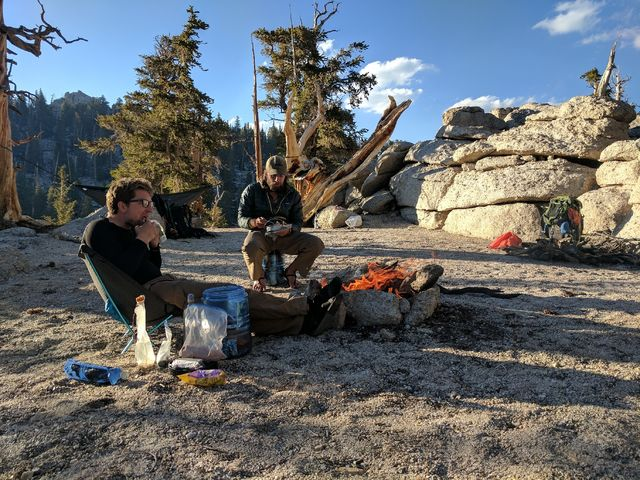# PCTself.__wrap_b=(t,n,e)=>{e=e||document.querySelector(`[data-br="\${t}"]`);let s=e.parentElement,r=R=>e.style.maxWidth=R+"px";e.style.maxWidth="";let o=s.clientWidth,i=s.clientHeight,c=o/2-.25,l=o+.5,u;if(o){for(;c+1<l;)u=Math.round((c+l)/2),r(u),s.clientHeight===i?l=u:c=u;r(l*n+o*(1-n))}e.__wrap_o||(e.__wrap_o=new ResizeObserver(()=>{self.__wrap_b(0,+e.dataset.brr,e)})).observe(s)};self.__wrap_b(":Rab6:",1)

A taste of what's to comeJun 03 2017

# No major snow so far...self.__wrap_b=(t,n,e)=>{e=e||document.querySelector(`[data-br="\${t}"]`);let s=e.parentElement,r=R=>e.style.maxWidth=R+"px";e.style.maxWidth="";let o=s.clientWidth,i=s.clientHeight,c=o/2-.25,l=o+.5,u;if(o){for(;c+1<l;)u=Math.round((c+l)/2),r(u),s.clientHeight===i?l=u:c=u;r(l*n+o*(1-n))}e.__wrap_o||(e.__wrap_o=new ResizeObserver(()=>{self.__wrap_b(0,+e.dataset.brr,e)})).observe(s)};self.__wrap_b(":Rmj6:",1)

Jun042017Tonight, for the second night in a row, we're camping above 10,000 feet. I don't know why, but that's neat to me.

It's been surprisingly good hiking -- not only have we not hit any major snow (no crampons required so far!) but the higher elevation hasn't really impacted anyone too badly. There's the obvious "this is harder to climb" effects, but no altitude sickness, headaches, dizziness, etc. Let's hope this keeps up!

And now, some pictures from today that I want to share just because:Hanging out by the fire, eating dinner, while waiting for the sun to go down (so we can go to bed)
First real Sierra day!Jun 05 2017